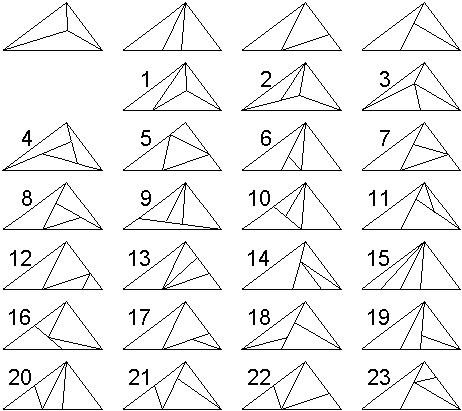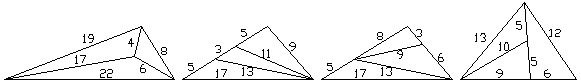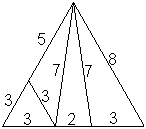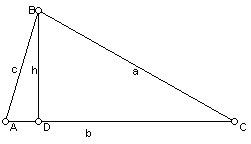TRIANGLES

An education in math should begin with the study of triangles.  If you're young, and have an option to take Geometry someday, I urge you to take the course.  You see, when you enter the real world, geometry is crucial in all types of businesses. For example, street signs, buildings and even marquees, all of these are things in the real world that come in triangular form and to be created and marketed successfully, the geometric aspect needs to be understood. Marquees and gazebos, especially can be geometrically intricate sometimes shaped as triangular prisms and other times more like square or rectangular prisms. Such students might think that I'd know everything about triangles.  Au contraire!  Mathematicians understand how little they understand.  From triangles to infinity, there is much left to know.

Triangle Partitions
To start, we'll cut triangles into smaller triangles.  Some definitions follow: Triangle Partition : A triangle divided into two or more smaller triangles. The number of triangles gives the order.Prime Triangle :  A triangle partition which contains no triangle partitions of lower order.  Prime Triangles are an idea of Marek Ctrnact.  Erich Friedman also worked on this problem.  The triangles below were found by Roger Phillips, Scott Purdy, Andrew Kennaugh, Matthew Cook, and Ed Pegg Jr.  Note that we are looking for triangles that are topologically distinct.  The order-6 prime triangles are probably incomplete.  They are hard to find.  The sequence so far:  1, 3, 8, 62, ??.  Roger Phillips enumerated the order-6 Prime triangles.  Dylan Thurston corrected a few errors and found a structure behind them.  If there are any duplicates, or missing triangles, please contact me.If we look beyond the prime triangles, how many different ways can a triangle be fractured into smaller triangles?  It seems to be an interesting puzzle in itself, and I'm not sure if I even have the level four triangle complete.  Is there a general scheme to these?  These triangles were originally discovered by Ignacio R, who found 20 order-4 triangles.  So did I.  Roger Phillips then pointed out triangles 1 and 16.  Bob Harris added triangle 10.  Are there any more order-4 triangles? Roger Phillips has found 181 order-5 triangles, which I have put on a page here.  Are any missing?  Any duplicates?  Miroslav Vicher noticed that 14=23.   The sequence so far 1, 4, 23, 180?, ???4 order-3 triangles, and 23 order-4 triangles.  Are any missing?

Another question to consider involves cutting a triangle into n parts with equal area.  In this question, topological distinction is no longer necessary.  A scalene triangle can be divided into 2 equal area triangular pieces in 3 ways.  It can be divided into 3 equal area triangular pieces in 16 ways.  The number of ways to divide a triangle into 4 equal area triangles is infinite, due to partition number 16, above.  Here are the 16 ways to divide a scalene triangle into 3 equal area triangular pieces.

Integer Triangles
Definition: An Integer Triangle is a triangle where all sides have integer length.  In such a triangle, cos A is a rational number -- a fraction. Here, you can see a number of integer triangles which share the same angles.  As it happens, a [7, 7, 13] triangle can be divided into two non-similar triangles with integer sides: [3, 5, 7] and [3, 7, 8].

It's easy to make spreadsheets for integer triangles.  You can use Excel, or the free StarOffice (www.sun.com).  For any rational number a, it is easy to find many integer triangles with an angle of arccos(a).  For example, here is a whole list of triangles with sides a b c for angle C equivalent to -1/n , where n = 2 to 21.  The angle C, opposite side c, is getting increasingly closer to being a right angle.

a  b  c cos[C]   a  b  c cos[C]   a  b  c cos[C]   a  b  c cos[C]
3  5  7  -1/2    3  7  8  -1/7    9 10 14  -1/12   5 17 18  -1/17
5  6  9  -1/3    2  2  3  -1/8   10 13 17  -1/13  15 24 29  -1/18
2  3  4  -1/4    3  6  7  -1/9    7  8 11  -1/14   8 19 21  -1/19
4  5  7  -1/5    5  7  9  -1/10   3  5  6  -1/15   5  6  8  -1/20
3  8  9  -1/6    6 11 13  -1/11   4 10 11  -1/16   9 14 17  -1/21
Minimal integer triangles of varying degrees

It turns out that integer triangles that have an angle in common are all closely related.  The simplest examples are the Pythagorean Triangles. Michael Somos has made a table of Pythagorean Triangles.  All are expressed by a formula in p and q, where a1 = q2-p2, a2 = 2*p*q, a3 = q2+p2.  a12 + a22 = a32, which gives a right triangle. Bill Harris has found a formula which will give infinite triangles with any given angle.

For what rational numbers a, b, and c will cos(arccos(a)+arccos(b)+arccos(c))=1 ?  This is a surprisingly deep question.  Here is an answer by William Watson. Marek Ctrnact verifies the first is minimal.William Watson wrote a program to solve the general problem.  Here are solutions to all order-4 triangle partitions, drawn to scale!

What is the smallest triangle, in terms of perimeter, that can be divided into 4 nonsimilar triangles with integer sides?  Bill Daly was intrigued by the problem.  Some of the methods Bill Daly used to solve the problem are here.  Konstantin Knop has found the minimal answer:Michael Somos has made a table of Heronian Triangles -- triangles with integer sides and integer area.  His Rational Triangles page is also related.

Daniel Scher's Geometry in Motion has many interactive problems with triangles.  A vaguely connected problem to all this is to divide a 45-degree right triangle into four pieces that can be rearranged into an equilateral triangle.  The solution is here.

Noam D. Elkies posted an interesting letter at rec.puzzles:  "There's a shortcut called Stewart's Theorem, for which we learned (at Stuyvesant Math Team -- those were the days) the mnemonic "a man and his dad put a bomb in the sink".  Misspell "sink" as "cinc", disemvowel the bomb and the cinc, and get Stewart's Theorem:  man + dad = bmb + cnc . Here a,b,c are sides of a triangle ABC; the "a" side is divided into m+n by a point at distance d from the opposite vertex.  m and n are labeled so that the products bmb and cnc involve disjoint line segments. In our case, we have: b=c=7, a=13, m=5, n=8.  So, 5*8*13 + 13 d^2 = 7^2 (8+5) = 49*13 So,  d^2 = 49 - 5*8 = 9 and d=3  as desired.

SOHCAHTOA and the Law of CosinesSOHCAHTOA   Sin(A) = Opposite/Hypotenuse   Cos(A) = Adjacent/Hypotenuse   Tan(A) = Opposite/Adjacent
The SOHCAHTOA mnemonic is one that everyone should know.  The Law of Cosine applies to more general triangles, though.In either triangle, CD = b - c cos A .  Also, h = c sin A .  From triangle BCD we have h2 = a2 - (CD)2 .  Combining all three of these, we obtain c2 sin2 A = a2 - (b - c cos A)2 = a2 - b2 + 2bc cos A - c2 cos2 A .  Solving for a2 from the first and third members, we obtain a2 = b2 + c2 (sin2 A + cos2 A) - 2bc cos A .  Since sin2 A + cos2A = 1, we obtain the following formula :
a2 = b2 + c2 - 2bc cos A
Which is the Law of Cosines.  By relabeling the triangle, different forms of the law can be obtained.  Solving for the angle, we obtainFrom Nick Baxter:  Find the smallest integer triangle where one angle is double the size of another.  Send Answer.  Bonus points for finding 3x, 4x, 5x, 6x, and 7x.

Can a triangular prism be fair?  Probably not, when tossed either glass or mud.  Here's related experimental data that Juha Saukkola pointed me to.